### Generalized Linear Mixed Model

In a generalized linear mixed model (GLMM) the G-side random effects are part of the linear predictor,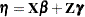, and the predictor is related nonlinearly to the conditional mean of the data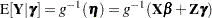where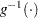is the inverse link funtion. The conditional distribution of the data, given the random effects, is a member of the exponential family of distributions, such as the binary, binomial, Poisson, gamma, beta, or chi-square distribution. Because the normal distribution is also a member of the exponential family, the class of the linear mixed models is a subset of the generalized linear mixed models. In order to completely specify a GLMM, you need to do the following:

1. Formulate the linear predictor, including fixed and random effects.

3. Choose the distribution of the response, conditional on the random effects, from the exponential family.

As an example, suppose thatpairs of twins are randomly selected in a matched-pair design. One of the twins in each pair receives a treatment and the outcome variable is some binary measure. This is a study withclusters (subjects) and each cluster is of size. If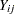denotes the binary response of twin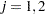in cluster, then a linear predictor for this experiment could be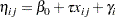where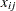denotes a regressor variable that takes on the valuefor the treated observation in each pair, and
otherwise. The
are pair-specific random effects that model heterogeneity across sets of twins and that induce a correlation between the members of each pair. By virtue of random sampling the sets of twins, it is reasonable to assume that theare independent and have equal variance. This leads to a diagonal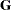matrix,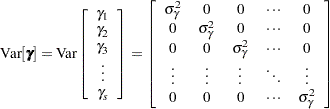A common link function for binary data is the logit link, which leads in the second step of model formulation to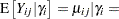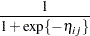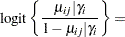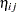The final step, choosing a distribution from the exponential family, is automatic in this example; only the binary distribution comes into play to model the distribution of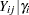.

As for the linear mixed model, there is a marginal model in the case of a generalized linear mixed model that results from integrating the joint distribution over the random effects. This marginal distribution is elusive for many GLMMs, and parameter estimation proceeds by either approximating the model or by approximating the marginal integral. Details of these approaches are described in the section Generalized Linear Mixed Models Theory, in Chapter 40, The GLIMMIX Procedure.

A marginal model, one that models correlation through the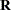matrix and does not involve G-side random effects, can also be formulated in the GLMM family; such models are the extension of the correlated-error models in the linear mixed model family. Because nonnormal distributions in the exponential family exhibit a functional mean-variance relationship, fully parametric estimation is not possible in such models. Instead, estimating equations are formed based on first-moment (mean) and second-moment (covariance) assumptions for the marginal data. The approaches for modeling correlated nonnormal data via generalized estimating equations (GEE) fall into this category (see, for example, Liang and Zeger 1986; Zeger and Liang 1986).